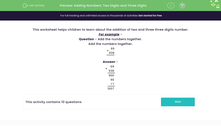# Add Two and Three-digit Numbers Using Knowledge of Place Value

In this worksheet, students will add together a two-digit number and a three digit number using their knowledge of place value.Key stage:  KS 2

Curriculum topic:   Number: Addition and Subtraction

Curriculum subtopic:   Add/Subtract up to Four-Digit Numbers

Difficulty level:#### Worksheet Overview

In this activity, we'll look at how to add two and three digits numbers together using our knowledge of place value.

Example:

Can you add the numbers below?

 + 6 9 9 3 8

So, how do we do this?

One method is to split them into hundreds, tens and ones before adding them.

1) First, we add the hundreds column: 900 + 0 = 900

2) Then, we add the tens column: 60 + 30 = 90

3) Next, we add the ones: 9 + 8 = 17

4) Finally, we add these together: 900 + 90 + 17 = 1,007

Remember to put 0s as placeholders.

Have a look below and you can see these steps written in number form.

 + 6 9 9 3 8 9 0 0 9 0 1 7 1 0 0 7

Ready to give this a go?### What is EdPlace?

We're your National Curriculum aligned online education content provider helping each child succeed in English, maths and science from year 1 to GCSE. With an EdPlace account you’ll be able to track and measure progress, helping each child achieve their best. We build confidence and attainment by personalising each child’s learning at a level that suits them.

Get started••••